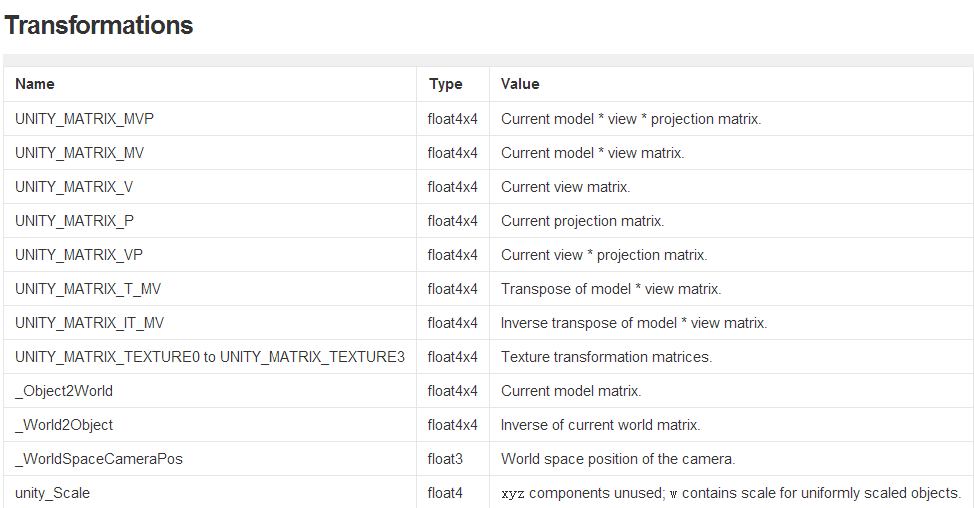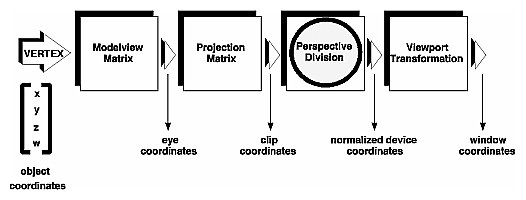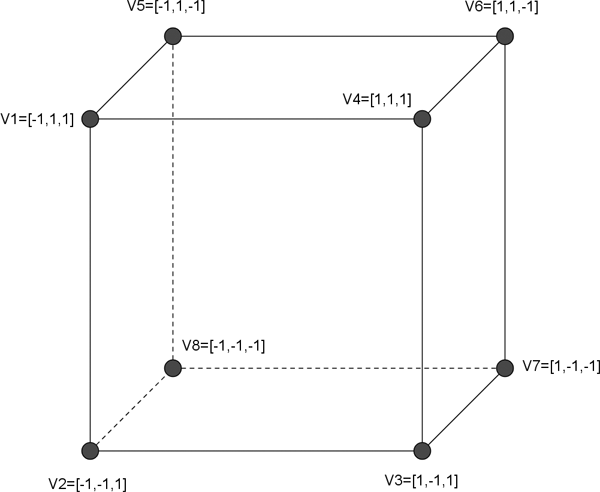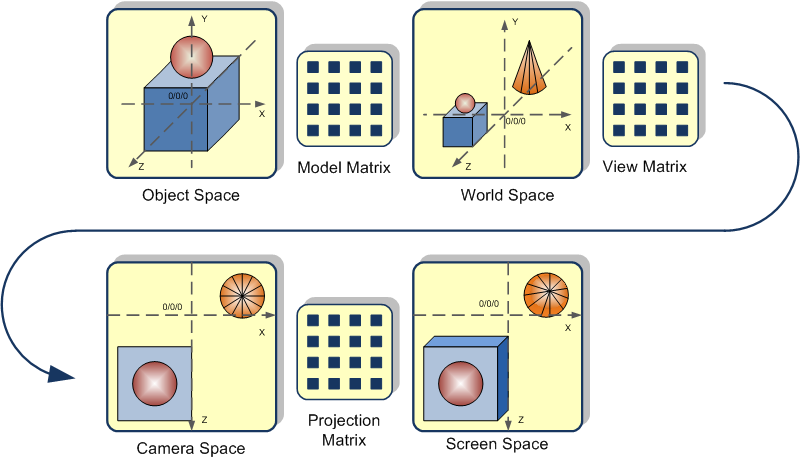# Unity Shader Matrix[Matrix]# 2、Matrix说明

## A、Stages of Vertex Transformation## B、The different Coordinate Systems in OpenGL

There are multiple coordinate Systems involved in 3D Graphics:

• Object Space
• World Space (aka Model Space)
• Camera Space (aka Eye Space or View Space)
• Screen Space (aka Clip Space)

So, what’s the difference between them?

Object Space

This is the local coordinate System of your Geometrical Objects. Imagine a Cube which consists of 8 vertices:When modelling this cube you do this in Object Space – the Object stands alone for itself, no other things in here.

World Space

This is the Space where everything is positioned. Imagine the Cube created above – it has been modelled in it’s own absolute Object Space. But you probably want the Cube at some other location in your World – so we need to transform the Object Coordinate System to the desired World coordinate System position. We do this using a Transformation Matrix – every vertex in the Cube (which is in Object Space) is multiplied by the World Matrix which transforms the vertex to it’s new position and orientation.

Let’s explain this with a example:

1. We have the Cube shown above
2. We want to position it in World coordinates at position X=5, Y=0, Z=0 (simply move it 5 units to the right)

What we need to do, is to multiply each vertex with the corresponding Model Matrix for the actual positioning of this object (we have different Model Matrices for differently located objects). The resulting Vertex has the new position in World Space.

Even if the math for doing the matrix multiplication is out of the scope i want to show you an example:

The Vector V2 is [-1,-1,1]. We have to use a 4 Component Vector (x,y,z,w) so we set w simply at 1 (-1,-1,1,1)
The Matrix M1 is:

[1,0,0,5,
0,1,0,0,
0,0,1,0,
0,0,0,1]

When we now multiply those two (M1 . V2) we get a new vector: [4,-1,1,1] – which is V2 moved 5 units to the left. This calculation is done for every vertex in the cube. If you don’t know how to actually do the matrix multiplication, just read one of the books mentioned at the bottom of this article (or the corresponding wikipedia article, your old math book, whatever, … :-))

Camera Space

Now that we have positioned everything in our World we want to look at it. First, there is no such “real” thing as a Camera in OpenGL. Think about the following for a short time:

• Moving a Video Camera Backward is the same as moving the filmed object forward

That’s exactly what we are going to do to get our view on the scene – multiply every vertex in the World Space with our View Matrix. Each Vertex is then in Camera Space – and the scene looks like we are looking at it through the camera.

Imagine the camera as a abstract thing which is on the positive Z-Axis and looks down the negative end of the Z-Axis. Imagine also a rotation and translation of the World around you so that you can see what you want to see – this rotation and translation is the View Matrix.

As for the World Space, the Mathematics for doing this is out of Scope for this Overview.

Projection Space

So, we have every vertex in a position that we see the scene the way we want it to see. The last Coordinate System transformation is from Camera to Screen Space – which is essentially nothing more than going from the 3D Coordinate System to the 2D Screen in front of us. This transformation is necessary as long as we don’t have real 3D Holographic Screens. In the process of transforming from Camera to Screen Space you can either choose a Orthographic or Perspective Projection.

The difference between those two is that the Orthographic Projection does not apply a perspective distortion and the perspective one does. Perspective Projection is the natural one as we Humans see in a perspective Way – things farther away from us appear smaller.

Graphical overview of the Transformation

If you never worked with 3D graphics before it’s probably more intuitive to take a look at the graphic below to get an idea on how the transformations actually modify the coordinates of the vertices:From top left to bottom right, the following actions occur:

1. Vertices of the Object to draw are in Object space (as modelled in your 3D Modeller)
2. … get transformed into World space by multiplying it with the Model Matrix
3. Vertices are now in World space (used to position the all the objects in your scene)
4. … get transformed into Camera space by multiplying it with the View Matrix
5. Vertices are now in View Space – think of it as if you were looking at the scene throught “the camera”
6. … get transformed into Screen space by multiplying it with the Projection Matrix
7. Vertex is now in Screen Space – This is actually what you see on your Display.

http://docs.unity3d.com/Manual/SL-BuiltinValues.html

http://www.glprogramming.com/red/chapter03.html

http://www.matrix44.net/cms/notes/opengl-3d-graphics/coordinate-systems-in-opengl